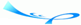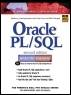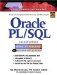# Chapter 8 Iterative Control

 Team-FlyOracle® PL/SQL® Interactive Workbook, Second EditionBy Benjamin Rosenzweig, Elena Silvestrova Table of Contents Appendix A.  Answers to Self-Review Questions

#### Lab 8.1 Self-Review Answers

A1:

Questions

Answers

Comments

1)

C

If there is no EXIT condition specified, a simple loop becomes an infinite loop. In other words, a sequence of statements will be executed an infinite number of times because there is no statement specifying when the loop must terminate.

A2:

Questions

Answers

Comments

2)

B

As soon as the EXIT statement is encountered, the loop is terminated.

A3:

Questions

Answers

Comments

3)

A

As long as the EXIT condition does not evaluate to TRUE, the control is never transferred to the EXIT statement. This will prevent a loop from terminating. For example,

` IF x > 5 THEN     EXIT;  END IF; `

In this case, the EXIT condition is a test condition of the IF statement. When the test condition of the IF statement evaluates to FALSE or NULL, the control is passed to the first executable statement after END IF.

A4:

Questions

Answers

Comments

4)

C

Once EXIT statement is executed, the control is transferred to the first executable statement after END LOOP.

A5:

Questions

Answers

Comments

5)

B

An EXIT condition of a simple loop is located inside the body of the loop. Therefore, the loop will always execute partly before the EXIT condition is evaluated.

#### Lab 8.2 Self-Review Answers

A1:

Questions

Answers

Comments

1)

A

Before a WHILE loop is executed, its test condition is evaluated. If the test condition yields FALSE, the WHILE loop is unable to execute.

A2:

Questions

Answers

Comments

2)

C

If a test condition always evaluates to TRUE, the WHILE loop is unable to terminate. As a result, it executes infinite number of times.

A3:

Questions

Answers

Comments

3)

B, C

A4:

Questions

Answers

Comments

4)

A

You will recall that a test condition must evaluate to FALSE or NULL for a WHILE loop to terminate. On the other hand, the EXIT condition must evaluate to TRUE. Thus, if the EXIT condition evaluates to TRUE before the test condition evaluates to FALSE, the WHILE loop terminates prematurely.

A5:

Questions

Answers

Comments

5)

A

If a test condition of a WHILE loop never evaluates to TRUE, the loop does not execute at all.

#### Lab 8.3 Self-Review Answers

A1:

Questions

Answers

Comments

1)

B

For the first iteration of the loop, the value of the loop counter is equal to the lower limit. For the second iteration of the loop, the value of the loop counter is implicitly incremented by 1. At this point, the value of the loop counter does not satisfy the range specified by the lower limit and the upper limit, so the loop terminates. For example,

` BEGIN     FOR i IN 1..1 LOOP        DBMS_OUTPUT.PUT_LINE ('i = '||i);     END LOOP;  END;  /  i = 1  PL/SQL procedure successfully completed. `

A2:

Questions

Answers

Comments

2)

A

The loop counter is unable to satisfy the range specified by the lower and upper limits.

A3:

Questions

Answers

Comments

3)

C

The loop counter is implicitly defined by the loop construct. As a result, it does not exist anywhere outside the loop.

A4:

Questions

Answers

Comments

4)

C

A5:

Questions

Answers

Comments

5)

B

The loop counter is initialized to the upper limit, and it is decremented by 1 for each iteration of the loop.

#### Lab 8.4 Self-Review Answers

A1:

Questions

Answers

Comments

1)

C

A2:

Questions

Answers

Comments

2)

B

Loop labels are optional feature and are used to improve readability.

A3:

Questions

Answers

Comments

3)

B

A4:

Questions

Answers

Comments

4)

B

It is considered bad programming practice to use the same name for different variables. When the same name is used for the loop counters, you are unable to reference the outer loop counter in the body of the inner loop. In order to differentiate between two variables having the same name, you must use loop labels when the variables are referenced.

A5:

Questions

Answers

Comments

5)

B

You must use loop labels only when outer and inner loop counters have the same name and you want to reference the outer loop counter in the inner loop. In other cases, it is not necessary to use a loop label when referencing the loop counter.

 Team-FlyTopOracle PL/SQL Interactive Workbook (2nd Edition)
ISBN: 0130473200
EAN: 2147483647
Year: 2002
Pages: 146

Similar book on Amazon

flylib.com © 2008-2017.
If you may any questions please contact us: flylib@qtcs.net